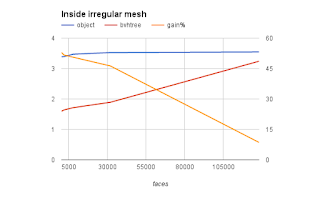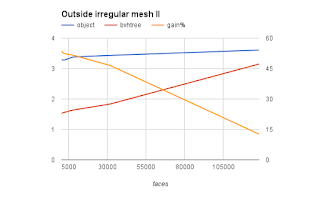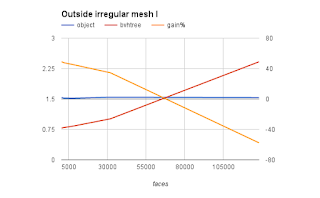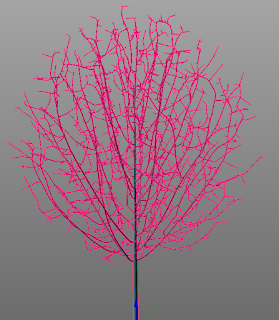### Performance of ray casting in Blender Python

The Python API for ray casts on objects and scenes was changed in a breaking manner in Blender 2.77. And because several of my add-ons depend on it, I not only had to fix them but I decided to have a look at the performance as well.
The central use case for ray casting in these add-ons is to check whether a point lies inside a mesh. This is determined by casting a ray and checking if the number of intersections is even or odd (where we count 0 as even). An even number of crossings means the point lies outside the mesh (true for the red points in the illustration) while an odd number means that the point lies inside the mesh (the green point). And the fun part is that this is true for any direction of the ray!

## Code

We can easily convert this to Python using Blenders API: given an object and point to test, we take some direction for our ray (here right along the x-direction) and check if there is an intersection. If so, we we test again but start at the intersection point, taking care to move a tiny bit along the ray cast direction to avoid getting 'stuck' at the intersection point due to numerical imprecision. We count the number of intersections and return True if this number is odd.

```import bpy
from mathutils import Vector
from mathutils.bvhtree import BVHTree

def q_inside(ob, point_in_object_space):
direction = Vector((1,0,0))
epsilon = direction * 1e-6
count = 0
result, point_in_object_space, normal, index = ob.ray_cast(point_in_object_space, direction)
while result:
count += 1
result, point_in_object_space, normal, index = ob.ray_cast(point_in_object_space + epsilon, direction)
return (count % 2) == 1
```

### Intersection with an object

We can now use this function to determine if a point lies within a mesh object:
```        q_inside(ob, (0,0,0))
```
Note that the point to check is in object space so if you want to check if a point in world space lies within the mesh you should convert it first using the inverted wordl matrix of the object.

### Intersection with a precalculated BVH tree

To determine if a ray hits any of the possibly millions of polygons that make up a mesh, the ray_cast() method internally constructs something called a BVH tree. This is a rather costly operation but fortunately a BVH tree is cached so when we perform many intersection tests this overhead should pay off. BVH trees however can also be constructed from a mesh object separately and offers exactly the same signature ray_cast() method. This means we can perform the same check to see if a point lies inside a mesh with following code:
```    sc = bpy.context.scene

bvhtree = BVHTree.FromObject(ob, sc)

q_inside(bvhtree, (0,0,0))```

## Performance

When doing millions of tests on the same mesh a small amount of overhead averages out so the question is if it is meaningful to create a separate BVH tree. If we look at the time it takes to do a million tests for meshes of different sizes we see that the overhead apparently is not the same but for larger meshes this difference loses significance:

### inside irregular mesh (subdivided Suzanne)

facesobjectbvhtreegain%
1280003.5473.2418.6
320003.5261.89246.3
80003.4731.71550.6
20003.3931.63951.7
5003.3911.59752.9### outside irregular mesh with mostly immediate initial hit

The initial data was for a point inside a mesh: such a point will always need at least two ray casts: the first will intersect some polygon, the second will veer off into infinity. If we test a point outside a mesh we sometimes will have only one ray cast and sometimes two. if we look at the latter scenario we see similar behavior:
facesobjectbvhtreegain%
1280003.6083.14812.7
320003.4341.83646.5
80003.3811.63751.6
20003.2771.55852.4
5003.2941.52853.6### outside irregular mesh with mostly no initial hits

A strange thing happens when the point to test is outside the mesh but the ray happens to point away from the mesh so that we will have zero intersections. Now for large meshes the overhead of a separate BVH tree appears larger!
facesobjectbvhtreegain%
1280001.5342.417-57.6
320001.5441.01134.5
80001.520.83245.2
20001.5230.79647.7
5001.5340.78349## Conclusion

i cannot quite explain the behavior of the ray_cast() performance for larger meshes when the point is outside the mesh and the test ray points away, but in arbitrary situations and moderately sized meshes a perfomance gain of 40% for the BVH tree is worthwhile when doing millions of tests.

### Floor boards add-on: another small feature

Again prompted by a question I added a feature to guarantee minimum plank lengths at the sides when using random plank lengths, to mimic real life behavior where you would not allow too short ends when laying a wooden floor.

In practice you would probably also try to avoid seams to line up in adjacent rows but if this happens you can easily switch to another random seed already.

The images below show the vertex color map (for clarity) with (left) and without a minimum offset of 12 cm, resulting in less short 'stubs'.## Other floor board articles

This blog already sports quite a number of articles on this add-on. The easiest way to see them all is to select the floor board tag (on the right, or click the link).

## the issue

imagine you are creating trees, either pure data structures or ones that try to mimic real trees, and that you are interested in the number of connected branch segments. How would you calculate these numbers for each node in the tree?
If we have a small tree like the one in the illustration, where we have placed the node index inside each sphere, we might store for each node the index of its parent, like in the list: `p = [ -1, 0, 1, 2, 2, 4 ]`Note that node number 0 has -1 as the index of its parent to indicate it has no parent because it is the root node. Also node 3 and 4 have the same parent node because they represent a fork in a branch.## naive solution

to calculate the number of connected nodes for each node in the tree we could simply iterate over all the nodes and traverse the list of parent nodes until we reach the root node, all the while adding 1 to the number of connections. This might look like this (the numbers are profiling information)
```      hits       time      t/hit

7385       4037      0.5   for p in parents:
683441    1627791      2.4    while p >= 0:
676057     410569      0.6     connections[p] += 1
676057     351262      0.5     p = parents[p]```
The result has the following values `c = [5, 4, 3, 0, 1, 0]`The tips have no connections while the root counts all nodes as connections minus itself so our 6-node tree had a root with 5 connections. This is also illustrated in the illustration (on the right).
If we time this simple algorithm for a moderately sized tree of just over 7000 nodes (which might look like the one in the image below to get a sense of the complexity) I find that it takes about 1.16 seconds on my machine.## numpy implementation

Now we all know that numpy is good at working with large arrays of numbers (and conveniently already bundled with Blender) so we might try some simple changes. Almost identical code but with numpy arrays instead of python lists:
```      hits       time      t/hit

7385       4563     0.6   for p in self.parent[:self.nverts]:
683441    1695443     2.5    while p >= 0:
676057    2004637     3.0     self.connections[p] += 1
676057     456995     0.7     p = self.parent[p] ```
But in fact this is slower! ( 2.68 seconds on my machine) If we look at the timings from the excellent kernprof line profiler (the numbers on the left of the code snippets) we see that numpy spends an awful lot of time on both the iteration over the parents and especially the indexing of the connection array. Apparently indexing a single element in a numpy array is slower than the equivalent operation on a python list. (There is a good explanation why indexing a single element of a numpy array takes so long on StackOverflow.)

## reworking the algorithm

It must be possible to do this faster right? Yes it is, have a look at the following code:
```      hits       time      t/hit

1           2      2.0   p = self.parent[:self.nverts]
1          19     19.0   p = p[p>=0]
137          97      0.7   while len(p):
136        1684     12.4    c = np.bincount(p)
136        1013      7.4    self.connections[:len(c)] += c
136        3395     25.0    p = self.parent[p]
136        1547     11.4    p = p[p>=0]```
This produces the same result (trust me, i'll explain in a minute), yet uses only milliseconds (7 to be precise). The trick is to use as much numpy code with looping and indexing built in as possible: `bincount()` counts the number of occurrences of a number and stores it the corresponding bin. So if 3 nodes have node 0 as their parent, c will be 3. Next we add this list in one go to the cumulative number of connections and finally we get the parent indices also in a single statement and remove the -1 entries (those nodes that reached root in their tree traversal). We repeat this as long as there are nodes that have not yet reached root.

## conclusion

Numpy might often be faster than pure Python but it pays to check, using careful profiling. Using an appropriate algorithm that does away as much as possible with any python overhead might however give you a sizeable speed improvement although the algorithm might not be immediately obvious to find.

## Space tree pro

This bit of research is not just academical, I am currently investigating options to speed up tree generation as implemented in my Space Tree Pro Blender add-on (available on BlenderMarket)